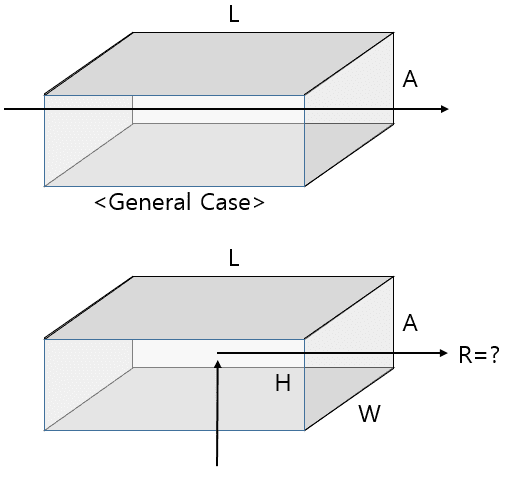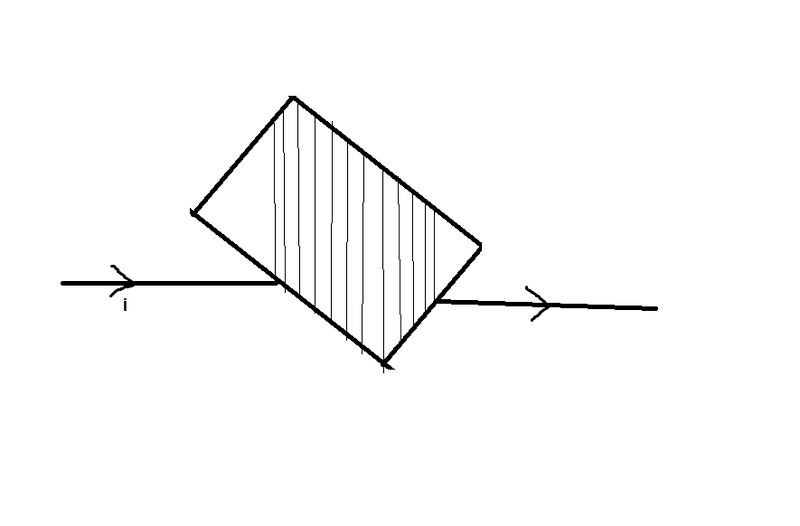# Resistance calculation on right angle current flow

As you know, resistance of a rectangular shape is given by R=ρ A/L

But how do we theoretically calculate the resistance of the bottom case? ( now, the current flow is not parrallel, current flows from bottom through the right side)

I would like to know if there is any references for this calculation,

As far, I found a reference on EDN forum, (http://www.edn.com/design/component...thod-to-quickly-estimate-PWB-trace-resistance)

Jaeger has experimentally calculated on a case which the current flows on right angle. (resistance drops by 0.56 *(sheet resistance) )

#### Attachments

•캡처.PNG
6.8 KB · Views: 453The cross-sectional area is to be perpendicular to the direction of current and the length is to be measured in the direction parallel to current flow.
So, in the second case, the cross-sectional area will be ##L\cdot W## and the length will be ##H##.
$$R=\frac{\rho\cdot Area}{Length}=\frac{\rho LW}{H}$$

Thanks arpon, but still your case is explaining when the current flow is vertical, I would like to know when the flow is turning to the right side. In other words, the two electrodes are on the bottom and the right side.

Last edited:In that case, you can break the resistance into several rectangular shaped resistances and then integrate.

nasu
Gold Member
Thanks arpon, but still your case is explaining when the current flow is vertical, I would like to know when the flow is turning to the right side. In other words, the two electrodes are on the bottom and the right side.
In that case you most likely will have to use some numerical calculations. Not likely to find and "easy" formula. The current density is not uniform in this case and you need to find how it depends on position.
Or you can experimentally research how the current varies with voltage and this will give you the resistance.

With how it seems, the integration would be a little more complicated, since currents usually flow throughout the entire conductor, something similar to what Arpon has suggested usually works. After all it depends on the cross sectional area. The expression of the shape itself is not hard to find, as to express it you could do with some plane equations.
Another method would be breaking the the shape into small resistors in parallel and integrate, but that'd be stupidly difficult I think.

nasu
Gold Member
I doubt it that it works at all. The fact that you put two electrodes on the faces does not mean that the current goes perpendicular to these slices.
Even if you have two "point "electrodes on the opposite faces the simple formula is only an approximation for an extended cube (rather than a thin wire).